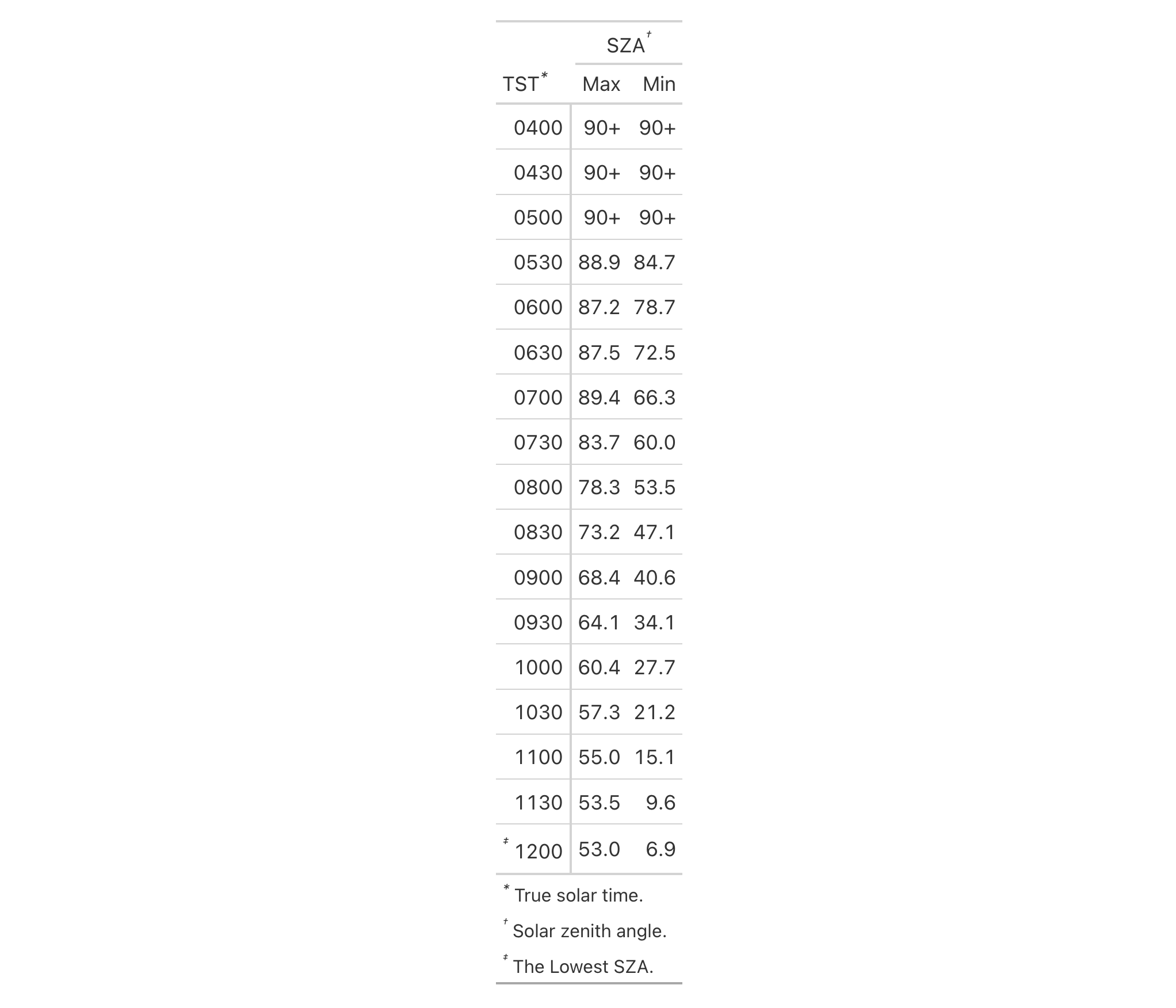Alter the footnote marks for any footnotes that may be present in the table. Either a vector of marks can be provided (including Unicode characters), or, a specific keyword could be used to signify a preset sequence. This function serves as a shortcut for using tab_options(footnotes.marks = {marks})

## Usage

opt_footnote_marks(data, marks)

## Arguments

data

A table object that is created using the gt() function.

marks

Either a character vector of length greater than 1 (that will represent the series of marks) or a single keyword that represents a preset sequence of marks. The valid keywords are: "numbers" (for numeric marks), "letters" and "LETTERS" (for lowercase and uppercase alphabetic marks), "standard" (for a traditional set of four symbol marks), and "extended" (which adds two more symbols to the standard set).

## Value

An object of class gt_tbl.

## Details

We can supply a vector of that will represent the series of marks. The series of footnote marks is recycled when its usage goes beyond the length of the set. At each cycle, the marks are simply doubled, tripled, and so on (e.g., * -> ** -> ***). The option exists for providing keywords for certain types of footnote marks. The keywords are:

• "numbers": numeric marks, they begin from 1 and these marks are not subject to recycling behavior

• "letters": miniscule alphabetic marks, internally uses the letters vector which contains 26 lowercase letters of the Roman alphabet

• "LETTERS": majuscule alphabetic marks, using the LETTERS vector which has 26 uppercase letters of the Roman alphabet

• "standard": symbolic marks, four symbols in total

• "extended": symbolic marks, extends the standard set by adding two more symbols, making six

## Examples

Use sza to create a gt table, adding three footnotes. Call opt_footnote_marks() to specify which footnote marks to use.

sza %>%
dplyr::filter(latitude == 30) %>%
dplyr::group_by(tst) %>%
dplyr::summarize(
SZA.Max = if (
all(is.na(sza))) {
NA
} else {
max(sza, na.rm = TRUE)
},
SZA.Min = if (
all(is.na(sza))) {
NA
} else {
min(sza, na.rm = TRUE)
},
.groups = "drop"
) %>%
gt(rowname_col = "tst") %>%
tab_spanner_delim(delim = ".") %>%
sub_missing(
columns = everything(),
missing_text = "90+"
) %>%
tab_footnote(
footnote = "True solar time.",
) %>%
tab_footnote(
footnote = "Solar zenith angle.",
locations = cells_column_spanners(
spanners = "spanner-SZA.Max"
)
) %>%
tab_footnote(
footnote = "The Lowest SZA.",
locations = cells_stub(rows = "1200")
) %>%
opt_footnote_marks(marks = "standard")## Function ID

9-1

Other table option functions: opt_align_table_header(), opt_all_caps(), opt_css(), opt_horizontal_padding(), opt_row_striping(), opt_stylize(), opt_table_font(), opt_table_lines(), opt_table_outline(), opt_vertical_padding()